# Unit circleIllustration of a unit circle. The variable t is an angle measure.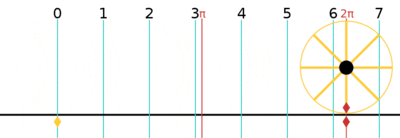Animation of the act of unrolling the circumference of a unit circle, a circle with radius of 1. Since C = 2πr, the circumference of a unit circle is .

In mathematics, a unit circle is a circle with a radius of one. Frequently, especially in trigonometry, the unit circle is the circle of radius one centered at the origin (0, 0) in the Cartesian coordinate system in the Euclidean plane. The unit circle is often denoted S1; the generalization to higher dimensions is the unit sphere.

If (x, y) is a point on the unit circle's circumference, then |x| and |y| are the lengths of the legs of a right triangle whose hypotenuse has length 1. Thus, by the Pythagorean theorem, x and y satisfy the equationSince x2 = (−x)2 for all x, and since the reflection of any point on the unit circle about the x- or y-axis is also on the unit circle, the above equation holds for all points (x, y) on the unit circle, not only those in the first quadrant.

The interior of the unit circle is called the open unit disk, while the interior of the unit circle combined with the unit circle itself is called the closed unit disk.

One may also use other notions of "distance" to define other "unit circles", such as the Riemannian circle; see the article on mathematical norms for additional examples.

## In the complex plane

The unit circle can be considered as the unit complex numbers, i.e., the set of complex numbers z of the formfor all t. This relation is Euler's formula. In quantum mechanics, this is referred to as phase factor.

## Trigonometric functions on the unit circle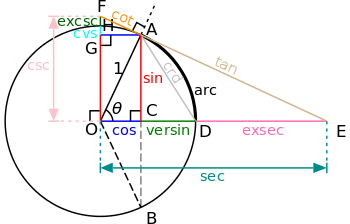All of the trigonometric functions of the angle θ (theta) can be constructed geometrically in terms of a unit circle centered at O.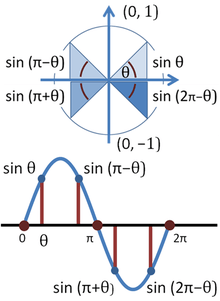Sine function on unit circle (top) and its graph (bottom)

The trigonometric functions cosine and sine of angle θ may be defined on the unit circle as follows: If (x, y) is a point on the unit circle, and if the ray from the origin (0, 0) to (x, y) makes an angle θ from the positive x-axis, (where counterclockwise turning is positive), thenThe equation x2 + y2 = 1 gives the relationThe unit circle also demonstrates that sine and cosine are periodic functions, with the identitiesfor any integer k.

Triangles constructed on the unit circle can also be used to illustrate the periodicity of the trigonometric functions. First, construct a radius OA from the origin to a point P(x1,y1) on the unit circle such that an angle t with 0 < t < π/2 is formed with the positive arm of the x-axis. Now consider a point Q(x1,0) and line segments PQ ⊥ OQ. The result is a right triangle △OPQ with ∠QOP = t. Because PQ has length y1, OQ length x1, and OA length 1, sin(t) = y1 and cos(t) = x1. Having established these equivalences, take another radius OR from the origin to a point R(−x1,y1) on the circle such that the same angle t is formed with the negative arm of the x-axis. Now consider a point S(−x1,0) and line segments RS ⊥ OS. The result is a right triangle △ORS with ∠SOR = t. It can hence be seen that, because ∠ROQ = π − t, R is at (cos(π − t),sin(π − t)) in the same way that P is at (cos(t),sin(t)). The conclusion is that, since (−x1,y1) is the same as (cos(π − t),sin(π − t)) and (x1,y1) is the same as (cos(t),sin(t)), it is true that sin(t) = sin(π − t) and −cos(t) = cos(π − t). It may be inferred in a similar manner that tan(π − t) = −tan(t), since tan(t) = y1/x1 and tan(π − t) = y1/x1. A simple demonstration of the above can be seen in the equality sin(π/4) = sin(/4) = 1/2.

When working with right triangles, sine, cosine, and other trigonometric functions only make sense for angle measures more than zero and less than π/2. However, when defined with the unit circle, these functions produce meaningful values for any real-valued angle measure – even those greater than 2π. In fact, all six standard trigonometric functions – sine, cosine, tangent, cotangent, secant, and cosecant, as well as archaic functions like versine and exsecant – can be defined geometrically in terms of a unit circle, as shown at right.

Using the unit circle, the values of any trigonometric function for many angles other than those labeled can be calculated without the use of a calculator by using the Sum and Difference Formulas.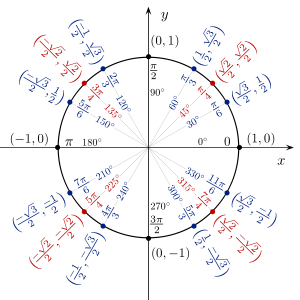The unit circle, showing coordinates of certain points

## Circle group

Main article: Circle group

Complex numbers can be identified with points in the Euclidean plane, namely the number a + bi is identified with the point (a, b). Under this identification, the unit circle is a group under multiplication, called the circle group. On the plane multiplication by cos θ + i sin θ gives a counterclockwise rotation by θ. This group has important applications in mathematics and science.

## Complex dynamics

Main article: Complex dynamics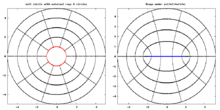Unit circle in complex dynamicsis a unit circle. It is a simplest case so it is widely used in study of dynamical systems.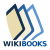Wikibooks has a book on the topic of: Trigonometry/The unit circleLook up unit circle in Wiktionary, the free dictionary.